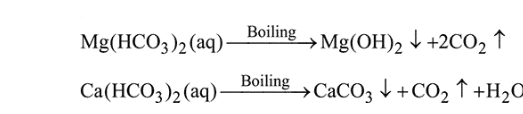# The temporary hardness of a water sample is due to compound X.

Question:

The temporary hardness of a water sample is due to compound $X$. Boiling this sample converts $X$ to compound Y. X and Y, respectively, are :

1. $\mathrm{Mg}\left(\mathrm{HCO}_{3}\right)_{2}$ and $\mathrm{Mg}(\mathrm{OH})_{2}$

2. $\mathrm{Ca}\left(\mathrm{HCO}_{3}\right)_{2}$ and $\mathrm{Ca}(\mathrm{OH})_{2}$

3. $\mathrm{Mg}\left(\mathrm{HCO}_{3}\right)_{2}$ and $\mathrm{MgCO}_{3}$

4. $\mathrm{Ca}\left(\mathrm{HCO}_{3}\right)_{2}$ and $\mathrm{CaO}$

Correct Option: 1

Solution:

Temporary hardness is caused by bicarbonates of calcium and magnesium. On boiling following changes occurs,